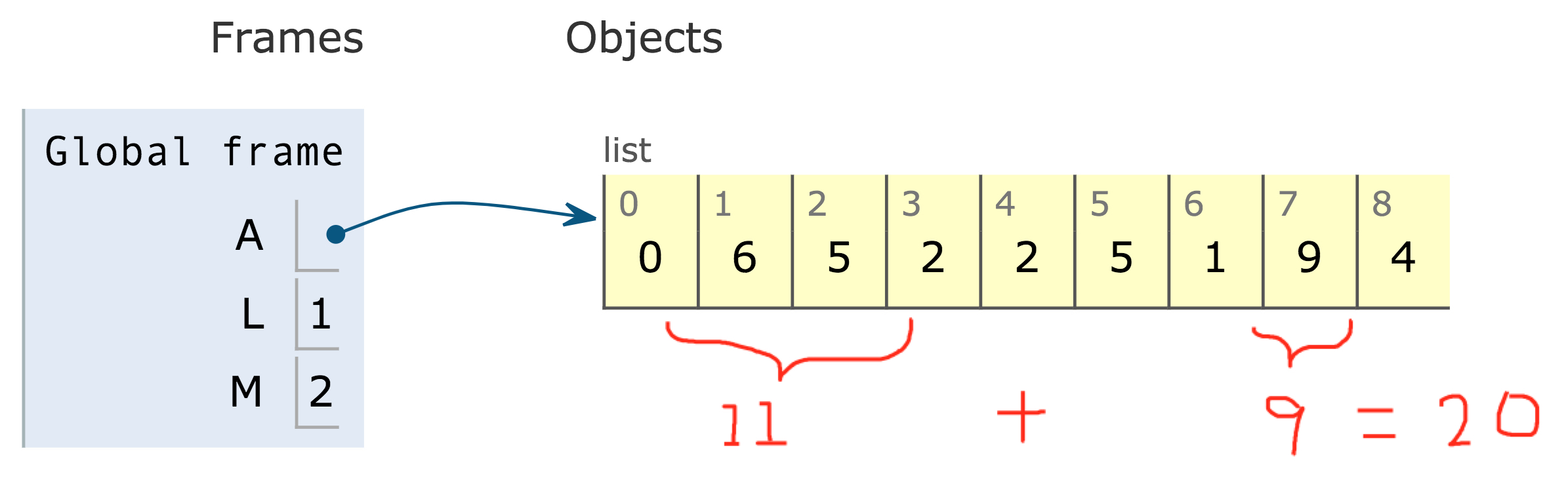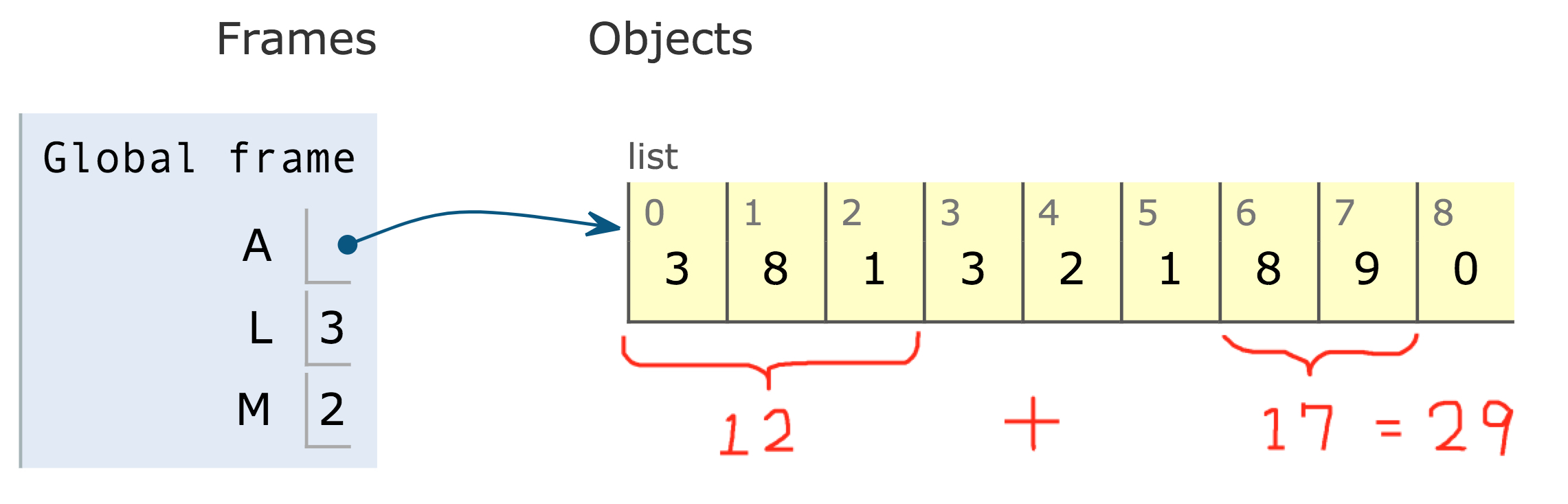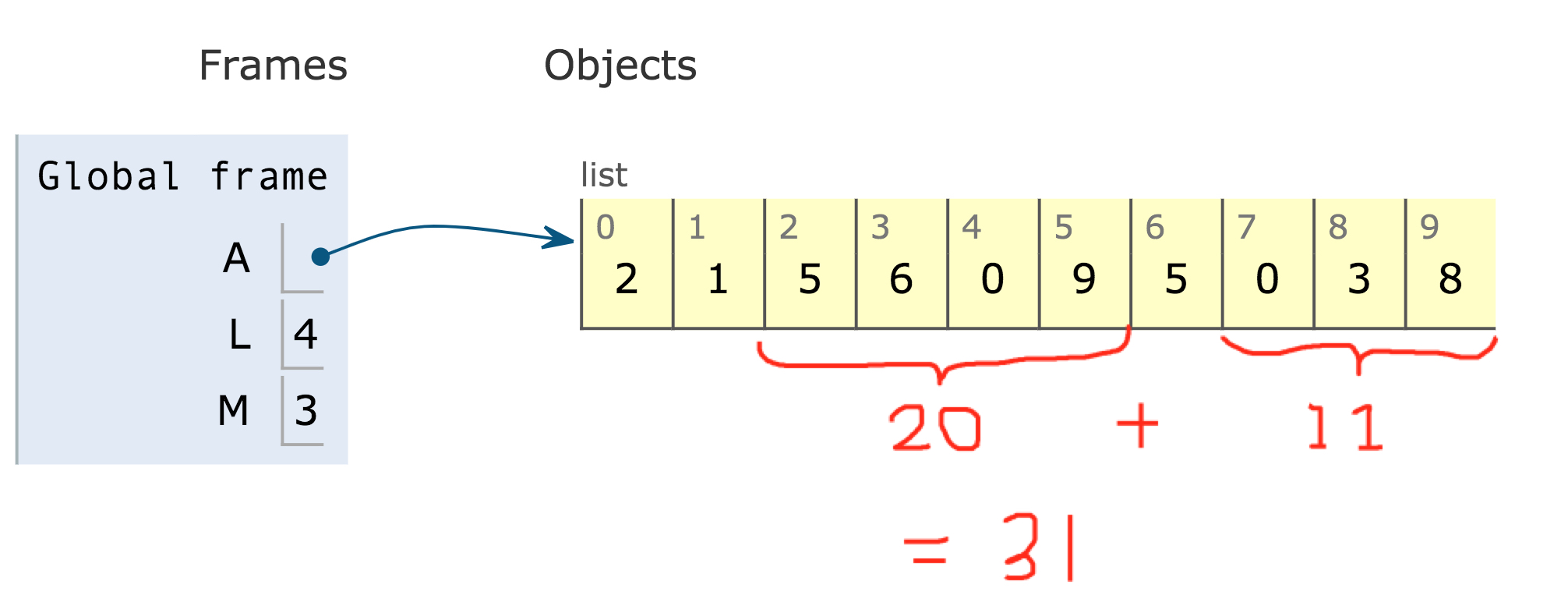# 1031. Maximum Sum of Two Non-Overlapping Subarrays

## Problem Setting¶

Given an array A of non-negative integers, return the maximum sum of elements in two non-overlapping (contiguous) subarrays, which have lengths L and M. (For clarification, the L-length subarray could occur before or after the M-length subarray.)

Formally, return the largest V for which V = (A[i] + A[i+1] + ... + A[i+L-1]) + (A[j] + A[j+1] + ... + A[j+M-1]) and either:

• 0 <= i < i + L - 1 < j < j + M - 1 < A.length, or
• 0 <= j < j + M - 1 < i < i + L - 1 < A.length.

### Example 1:¶

Input: A = [0,6,5,2,2,5,1,9,4], L = 1, M = 2

Output: 20

Explanation: One choice of subarrays is  with length 1, and [6,5] with length 2.### Example 2:¶

Input: A = [3,8,1,3,2,1,8,9,0], L = 3, M = 2

Output: 29

Explanation: One choice of subarrays is [3,8,1] with length 3, and [8,9] with length 2.### Example 3:¶

Input: A = [2,1,5,6,0,9,5,0,3,8], L = 4, M = 3

Output: 31

Explanation: One choice of subarrays is [5,6,0,9] with length 4, and [3,8] with length 3.## Solution¶

This problem can be solved by dynamic programming approach where

### Solution from lee215¶

This solution is $O(N)$ time and $O(1)$ space complexity.

In :
class Solution(object):
def maxSumTwoNoOverlap(self, A, L, M):
for i in range(1, len(A)): # xrange -> range by Hiro
A[i] += A[i - 1]
res, Lmax, Mmax = A[L + M - 1], A[L - 1], A[M - 1]
for i in range(L + M, len(A)): # xrange -> range by Hiro
Lmax = max(Lmax, A[i - M] - A[i - L - M])
Mmax = max(Mmax, A[i - L] - A[i - L - M])
res = max(res, Lmax + A[i] - A[i - M], Mmax + A[i] - A[i - L])
return res


### Execution¶

In :
A = [0,6,5,2,2,5,1,9,4]; L = 1; M = 2
s = Solution()
s.maxSumTwoNoOverlap(A, L, M)

Out:
20

### Step-by-step¶

L3-4: the first loop creates cumulative sum of list A.

#### Highlight¶

This list of cumulative sum of elements are used to calculate the contiguous n elements by A[i - n] - A[i].

In :
A = [0,6,5,2,2,5,1,9,4]
A

Out:
[0, 6, 5, 2, 2, 5, 1, 9, 4]
In :
for i in range(1, len(A)): # xrange -> range by Hiro
A[i] += A[i - 1]
A

Out:
[0, 6, 11, 13, 15, 20, 21, 30, 34]

L5:

• res: initialize the variable for result
• Lmax: Ls elements of the cumulative list i.e., 0 in the example 1
• Mmax: M's elements of the cumulative list i.e., 6 in the example 1
In :
res, Lmax, Mmax = A[L + M - 1], A[L - 1], A[M - 1]
res, Lmax, Mmax

Out:
(11, 0, 6)

L6: the second loop starts from L+M to the end of the list.

• Lmax is the case when L contiguous elements are taken first
• Mmax is the case when M contiguous elements are taken first

Finally, it compares 2 cases (L elements first taken or M elements first taken) including the existing maximum result res.

In :
res = A[L + M - 1]
for i in range(L + M, len(A)):
print('='*40)
print('processing at {}th element'.format(i))
print('{} [{}] {}'.format(A[:i], A[i], A[i+1:]) )
Lmax = max(Lmax, A[i - M] - A[i - L - M])
Mmax = max(Mmax, A[i - L] - A[i - L - M])
res = max(res, Lmax + A[i] - A[i - M], Mmax + A[i] - A[i - L])

print('Lmax:', Lmax)
print('Mmax:', Mmax)
print('A[i] - A[i - M]:', A[i] - A[i - M])
print('A[i] - A[i - L]:', A[i] - A[i - L])

print('Current maximum result:', res)


========================================
processing at 3th element
[0, 6, 11]  [15, 20, 21, 30, 34]
Lmax: 6
Mmax: 11
A[i] - A[i - M]: 7
A[i] - A[i - L]: 2
Current maximum result: 13
========================================
processing at 4th element
[0, 6, 11, 13]  [20, 21, 30, 34]
Lmax: 6
Mmax: 11
A[i] - A[i - M]: 4
A[i] - A[i - L]: 2
Current maximum result: 13
========================================
processing at 5th element
[0, 6, 11, 13, 15]  [21, 30, 34]
Lmax: 6
Mmax: 11
A[i] - A[i - M]: 7
A[i] - A[i - L]: 5
Current maximum result: 16
========================================
processing at 6th element
[0, 6, 11, 13, 15, 20]  [30, 34]
Lmax: 6
Mmax: 11
A[i] - A[i - M]: 6
A[i] - A[i - L]: 1
Current maximum result: 16
========================================
processing at 7th element
[0, 6, 11, 13, 15, 20, 21]  
Lmax: 6
Mmax: 11
A[i] - A[i - M]: 10
A[i] - A[i - L]: 9
Current maximum result: 20
========================================
processing at 8th element
[0, 6, 11, 13, 15, 20, 21, 30]  []
Lmax: 6
Mmax: 11
A[i] - A[i - M]: 13
A[i] - A[i - L]: 4
Current maximum result: 20